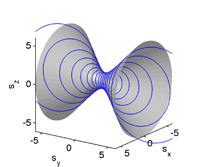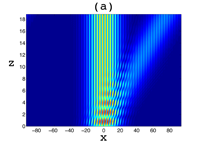## Classical and quantum dynamics in the (non-Hermitian) Swanson oscillator

Eva-Maria Graefe, Hans Jürgen Korsch, Alexander Rush, Roman Schubert

The non-Hermitian quadratic oscillator studied by Swanson is one of the popular PT-symmetric model systems. Here a full classical description of its dynamics is derived using recently developed metriplectic flow equations, which combine the classical symplectic flow for Hermitian systems with a dissipative metric flow for the anti-Hermitian part. Closed form expressions for the metric and phase-space trajectories are presented which are found to be periodic in time. Since the Hamiltonian is only quadratic the classical dynamics exactly describes the quantum dynamics of Gaussian wave packets. It is shown that the classical metric and trajectories as well as the quantum wave functions can diverge in finite time even though the PT-symmetry is unbroken, i.e., the eigenvalues are purely real.

http://arxiv.org/abs/1409.6456
Quantum Physics (quant-ph); Mathematical Physics (math-ph)

## Information Geometry of Complex Hamiltonians and Exceptional Points

Dorje C. Brody, Eva-Maria Graefe

Information geometry provides a tool to systematically investigate parameter sensitivity of the state of a system. If a physical system is described by a linear combination of eigenstates of a complex (that is, non-Hermitian) Hamiltonian, then there can be phase transitions where dynamical properties of the system change abruptly. In the vicinities of the transition points, the state of the system becomes highly sensitive to the changes of the parameters in the Hamiltonian. The parameter sensitivity can then be measured in terms of the Fisher-Rao metric and the associated curvature of the parameter-space manifold. A general scheme for the geometric study of parameter-space manifolds of eigenstates of complex Hamiltonians is outlined here, leading to generic expressions for the metric.

http://arxiv.org/abs/1307.4017
Quantum Physics (quant-ph); Statistical Mechanics (cond-mat.stat-mech); Mathematical Physics (math-ph)

## Mean-field approximation for a Bose-Hubbard dimer with complex interaction strength

Eva-Maria Graefe, Chiara Liverani

A generalised mean-field approximation for non-Hermitian many-particle systems has been introduced recently for a Bose-Hubbard dimer with complex on-site energies. Here we apply this approximation to a Bose-Hubbard dimer with a complex particle interaction term, modelling losses due to interactions in a two mode Bose-Einstein condensate. We derive the mean-field equations of motion leading to nonlinear dissipative Bloch dynamics, related to a nontrivial complex generalisation of the nonlinear Schrodinger equation. It is shown that depending on the parameter values there can be up to six stationary states. Further, for small values of the interaction strength the dynamics shows limit cycles.

http://arxiv.org/abs/1305.7160
Quantum Physics (quant-ph); Mathematical Physics (math-ph)

## Mixed-state evolution in the presence of gain and loss

Dorje C. Brody, Eva-Maria Graefe

A model is proposed that describes the evolution of a mixed state of a quantum system for which gain and loss of energy or amplitude are present. Properties of the model are worked out in detail. In particular, invariant subspaces of the space of density matrices corresponding to the fixed points of the dynamics are identified, and the existence of a transition between the phase in which gain and loss are balanced and the phase in which this balance is lost is illustrated in terms of the time average of observables. The model is extended to include a noise term that results from a uniform random perturbation generated by white noise. Numerical studies of example systems show the emergence of equilibrium states that suppress the phase transition.

http://arxiv.org/abs/1208.5297

Quantum Physics (quant-ph); Mathematical Physics (math-ph)

## Breakdown of adiabatic transfer schemes in the presence of decay

Eva-Maria Graefe, Alexei A. Mailybaev, Nimrod Moiseyev

In atomic physics, adiabatic evolution is often used to achieve a robust and efficient population transfer. Many adiabatic schemes have also been implemented in optical waveguide structures. Recently there has been increasing interests in the influence of decay and absorption, and their engineering applications. Here it is shown that contrary to what is often assumed, even a small decay can significantly influence the dynamical behaviour of a system, above and beyond a mere change of the overall norm. In particular, a small decay can lead to a breakdown of adiabatic transfer schemes, even when both the spectrum and the eigenfunctions are only sightly modified. This is demonstrated for the decaying version of a STIRAP scheme that has recently been implemented in optical waveguide structures. It is found that the transfer property of the scheme breaks down at a sharp threshold, which can be estimated by simple analytical arguments.

http://arxiv.org/abs/1207.5235
Quantum Physics (quant-ph); Mathematical Physics (math-ph); Optics (physics.optics)

## Stationary states of a PT-symmetric two-mode Bose-Einstein condensate

Eva-Maria Graefe

The understanding of nonlinear PT-symmetric quantum systems, arising for example in the theory of Bose-Einstein condensates in PT-symmetric potentials, is widely based on numerical investigations, and little is known about generic features induced by the interplay of PT-symmetry and nonlinearity. To gain deeper insights it is important to have analytically solvable toy-models at hand. In the present paper the stationary states of a simple toy-model of a PT-symmetric system are investigated. The model can be interpreted as a simple description of a Bose-Einstein condensate in a PT-symmetric double well trap in a two-mode approximation. The eigenvalues and eigenstates of the system can be explicitly calculated in a straight forward manner; the resulting structures resemble those that have recently been found numerically for a more realistic PT-symmetric double delta potential. In addition, a continuation of the system is introduced that allows an interpretation in terms of a simple linear matrix model.

http://arxiv.org/abs/1206.4806
Quantum Physics (quant-ph); Quantum Gases (cond-mat.quant-gas)

## Complexified coherent states and quantum evolution with non-Hermitian Hamiltonians

Eva-Maria Graefe, Roman Schubert

The complex geometry underlying the Schr\”odinger dynamics of coherent states for non-Hermitian Hamiltonians is investigated. In particular two seemingly contradictory approaches are compared: (i) a complex WKB formalism, for which the centres of coherent states naturally evolve along complex trajectories, which leads to a class of complexified coherent states; (ii) the investigation of the dynamical equations for the real expectation values of position and momentum, for which an Ehrenfest theorem has been derived in a previous paper, yielding real but non-Hamiltonian classical dynamics on phase space for the real centres of coherent states. Both approaches become exact for quadratic Hamiltonians. The apparent contradiction is resolved building on an observation by Huber, Heller and Littlejohn, that complexified coherent states are equivalent if their centres lie on a specific complex Lagrangian manifold. A rich underlying complex symplectic geometry is unravelled. In particular a natural complex structure is identified that defines a projection from complex to real phase space, mapping complexified coherent states to their real equivalents.

http://arxiv.org/abs/1111.1877
Mathematical Physics (math-ph); Quantum Physics (quant-ph)

## Quantum signatures of three coalescing eigenfunctions

Gilles Demange, Eva-Maria Graefe

Parameter dependent non-Hermitian quantum systems typically not only possess eigenvalue degeneracies, but also degeneracies of the corresponding eigenfunctions at exceptional points. While the effect of two coalescing eigenfunctions on cyclic parameter variation is well investigated, little attention has hitherto been paid to the effect of more than two coalescing eigenfunctions. Here a characterisation of behaviours of symmetric Hamiltonians with three coalescing eigenfunctions is presented, using perturbation theory for non-Hermitian operators. Two main types of parameter perturbations need to be distinguished, which lead to characteristic eigenvalue and eigenvector patterns under cyclic variation. A physical system is introduced for which both behaviours might be experimentally accessible.

http://arxiv.org/abs/1110.1489
Quantum Physics (quant-ph); Mathematical Physics (math-ph)

## Coquaternionic quantum dynamics for two-level systems

Dorje C. Brody, Eva-Maria GraefeThe dynamical aspects of a spin-1/2 particle in Hermitian coquaternionic quantum theory is investigated. It is shown that the time evolution exhibits three different characteristics, depending on the values of the parameters of the Hamiltonian. When energy eigenvalues are real, the evolution is either isomorphic to that of a complex Hermitian theory on a spherical state space, or else it remains unitary along an open orbit on a hyperbolic state space. When energy eigenvalues form a complex conjugate pair, the orbit of the time evolution closes again even though the state space is hyperbolic.

http://arxiv.org/abs/1105.4038
Mathematical Physics (math-ph); Quantum Physics (quant-ph)

## PT-Symmetric Sinusoidal Optical Lattices at the Symmetry-Breaking Threshold

Eva-Maria Graefe, H. F. JonesThe PT-symmetric potential $$V_0[\cos(2\pi x/a)+i\lambda\sin(2\pi x/a)]$$ has a completely real spectrum for $$\lambda\le 1$$, and begins to develop complex eigenvalues for $$\lambda>1$$. At the symmetry-breaking threshold $$\lambda=1$$ some of the eigenvectors become degenerate, giving rise to a Jordan-block structure for each degenerate eigenvector. In general this is expected to result in a secular growth in the amplitude of the wave. However, it has been shown in a recent paper by Longhi, by numerical simulation and by the use of perturbation theory, that for a broad initial wave packet this growth is suppressed, and instead a saturation leading to a constant maximum amplitude is observed. We revisit this problem by explicitly constructing the Bloch wave-functions and the associated Jordan functions and using the method of stationary states to find the dependence on the longitudinal distance $$z$$ for a variety of different initial wave packets. This allows us to show in detail how the saturation of the linear growth arises from the close connection between the contributions of the Jordan functions and those of the neighbouring Bloch waves.

http://arxiv.org/abs/1104.2838
Optics (physics.optics); Quantum Physics (quant-ph)